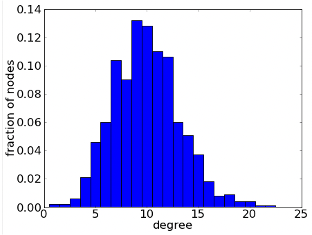# Graph Theory

## Exercise 1

1. Open Matlab and load the file ‘matrix.mat’. This is an fMRI correlation matrix, with 200 brain regions
2. Try plotting the matrix using the command: imagesc(matrix). What do you notice about it?
3. Using the script ‘exercise_1.m’, plot the correlation distribution for the correlation matrix.

## Exercise 1

1. Threshold and binarise the matrix at 10% density (see if you can understand exactly what the code is doing and why! We often binarise networks to remove weak ‘noisy’ correlations).
2. Plot the degree distribution of the network. Remember the degree distribution is P(k), i.e. the probability that a node has degree k (see figure).## Exercise 1

1. Using the BCT toolbox, calculate the mean shortest path length $L$ and the clustering coefficient $C$, for your thresholded, binarized network.
Hint: the BCT function to calculate clustering coefficient begins with: clustering_coef_…
The function to calculate the average shortest path length is ‘charpath.m’. But be warned - charpath.m doesn’t take the input you might expect. Read the documentation carefully!
• Discuss: What do you think are the pros/cons of binarizing a network vs using the fully weighted version?

# Exercise 2

Continue here after returning from main room

## Exercise 2

1. Read the BCT’s section on null networks here
2. We want to assess whether the $C$ and $L$ we obtained earlier are higher or lower than we would expect in random networks. Select the appropriate null network model from the BCT (preserving degree distribution) and use it to generate 100 null networks in Matlab.

## Exercise 2

1. Calculate the mean $C$ and $L$ for each of these null networks ($C_{r}$ and $L_{r}$)
2. Networks with $\sigma = \frac{C/C_{r}}{L/L_{r}} > 1$ are often defined as small-world (high clustering and short path length). Is our brain network small-world? You can learn more about small-world networks here